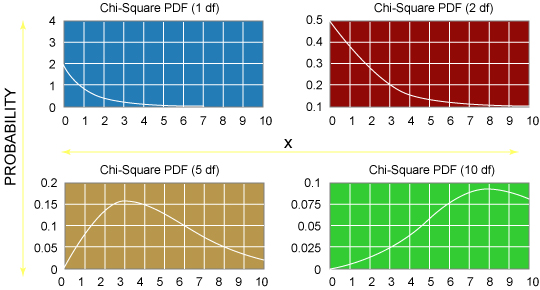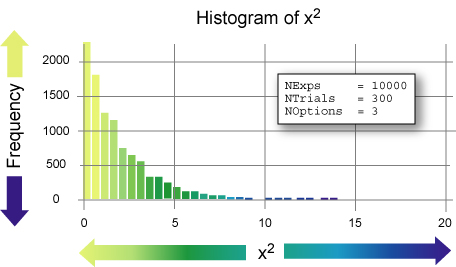Search

Useful Lists

Partners

Online Manuals

Take Web Data Analysis To The Next Level With PHP
By Paul Meagher - 2003-12-22 Page:  1 2 3 4 5 6 7 8 9 10 11 12 13

## Look At The Chi Square Sampling Distribution

(The reference image for the following graphic comes from the online NIST/SEMATECH Engineering Statistics Internet Handbook.)

Figure 2. Chi Square graphsIn each of the graphs, the bottom axis reflects the size of an obtained Chi Square score (range showing is 0 to 10). The left axis shows the probability, or relative frequency of occurrence, of various Chi Square values.

As you study these Chi Square graphs, note that the shape of the probability functions change when you vary the degrees of freedom, or df, in your experiment. In the case of poll data, the degrees of freedom is computed by noting the number of response options in the poll (k) and subtracting 1 from that value (df = k - 1).

In general, the probability of obtaining a large Chi Square value goes down as you increase the number of response options in your study. This is because as you add response options, you increase the number of squared difference scores -- (Observed - Expected)2 -- you can sum over. So, as you add response options, the statistical probability of obtaining a large Chi Square value should increase and the probability of obtaining smaller Chi Square value decreases. This is why the shape of the Chi Square sampling distribution changes for different df values.

Also, note that you are generally not interested in the point probability of the Chi Square outcome, but rather are interested in the summed area of the curve falling to the right of the obtained value. This tail probability tells you whether obtaining a value as extreme as the one you observe is likely (such as a large tail area) or not (a small tail area). (In practice, I don't use such graphs to compute tail probabilities because I can implement mathematical functions to return the tail probability for a given Chi Square value. This is performed in the Chi Square program that I discuss later in this article.)

To gain further insight into how these graphs were derived, look at how you can simulate the contents of the graph corresponding to df = 2 (which implies k = 3). Imagine putting the numbers 1, 2, and 3 in a hat, shaking it, selecting a number, and recording the selected number for that trial. Run this experiment for 300 trials and compute the frequencies at which 1, 2, and 3 occur.

Each time you run this experiment you should expect a slightly different frequency distribution for the outcomes that reflects sampling variability and is not a real bias among the response alternatives.

The Multinomial class that follows implements this idea. You initialize the class with values indicating the number of experiments you want to run, the number of trials per experiment, and the number of options per trial. The outcome of each experiment is recorded in an array called `Outcomes`.

Listing 1. Multinomial class outcomes
 `````` NExps = \$NExps; \$this->NTrials = \$NTrials; \$this->NOptions = \$NOptions; for (\$i=0; \$i < \$this->NExps; \$i++) { \$this->Outcomes[\$i] = \$this->runExperiment(); } } function runExperiment() { \$Outcome = array(); for (\$i = 0; \$i < \$this->NExps; \$i++){ \$choice = rand(1,\$this->NOptions); \$Outcome[\$choice]++; } return \$Outcome; } } ?> ``````

Note that the `runExperiment` method is the critical part of the script and implements the random choice of a response alternative and keeps track of which choices have been made so far in the simulated experiment.

To find the sampling distribution of the Chi Square statistic, simply take the outcome of each experiment and compute a Chi Square statistic for that result. This Chi Square statistic will vary from experiment to experiment due to random sampling variability.

The following script writes the obtained Chi Square statistic from each experiment to an output file for later plotting.

Listing 2. Writing the obtained Chi Square statistic to output file
 `````` Outcomes[\$i]); // Load obtained chi square value into sampling distribution array \$distribution[\$i] = \$chi->ChiSqObt; // Write obtained chi square value to file fputs(\$output, \$distribution[\$i]."\n"); } fclose (\$output); ?> ``````

To visualize the results expected from running this experiment, the simplest route for me was to load the `data.txt` file into the open source statistics package R, run the histogram command, and edit the plot in a graphics editor, as in the following:

 `````` x = scan("data.txt") hist(x, 50) ``````

As you can see, the histogram of these Chi Square values approximate the continuous Chi Square distribution presented above for df = 2.

Figure 3. Values approximate continuous distribution for df = 2In the next few sections, I focus on explaining how the Chi Square software used in this simulation works. Ordinarily the Chi Square software would be used to analyze real nominal data (such as Web poll results, weekly traffic reports, or customer brand-preference reports) instead of the simulated data you used. You might also be interested in other outputs that the software generates, such as summary tables and tail probability.

View Take Web Data Analysis To The Next Level With PHP Discussion

Page:  1 2 3 4 5 6 7 8 9 10 11 12 13 Next Page: Chi Square Instance Variables